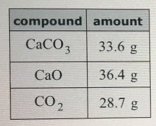# Problem: Calcium carbonate decomposes to form calcium oxide and carbon dioxide, like this:CaCO3(s) → CaO(s) + CO2(g)At a certain temperature, a chemist finds that a 6.1 L reaction vessel containing a mixture of calcium carbonate, calcium oxide, and carbon dioxide at equilibrium has the following composition:Calculate the value of the equilibrium constant Kc for this reaction. Round your answer to 2 significant figures.

###### FREE Expert Solution

We’re being asked to determine the equilibrium constant  for this reaction:

CaCO3(s) → CaO(s) + CO2(g)

Recall that the equilibrium constant is the ratio of the products and reactants

We use Kp when dealing with pressure and Kc when dealing with concentration:

$\overline{){{\mathbf{K}}}_{{\mathbf{p}}}{\mathbf{=}}\frac{{\mathbf{P}}_{\mathbf{products}}}{{\mathbf{P}}_{\mathbf{reactants}}}}$     $\overline{){{\mathbf{K}}}_{{\mathbf{c}}}{\mathbf{=}}\frac{\mathbf{\left[}\mathbf{products}\mathbf{\right]}}{\mathbf{\left[}\mathbf{reactants}\mathbf{\right]}}}$

Note that solid and liquid compounds are ignored in the equilibrium expression.

85% (50 ratings)###### Problem Details

Calcium carbonate decomposes to form calcium oxide and carbon dioxide, like this:

CaCO3(s) → CaO(s) + CO2(g)

At a certain temperature, a chemist finds that a 6.1 L reaction vessel containing a mixture of calcium carbonate, calcium oxide, and carbon dioxide at equilibrium has the following composition:Calculate the value of the equilibrium constant Kc for this reaction. Round your answer to 2 significant figures.